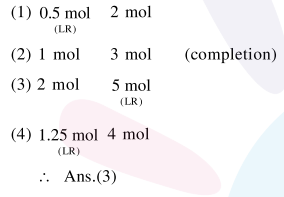Deepak Scored 45->99%ile with Bounce Back Crack Course. You can do it too!

# For a reaction,

Question:

For a reaction,

$\mathrm{N}_{2}(\mathrm{~g})+3 \mathrm{H}_{2}(\mathrm{~g}) \rightarrow 2 \mathrm{NH}_{3}(\mathrm{~g})$

identify dihydrogen $\left(\mathrm{H}_{2}\right)$ as a limiting reagent in the following reaction mixtures.

1. $14 \mathrm{~g}$ of $\mathrm{N}_{2}+4 \mathrm{~g}$ of $\mathrm{H}_{2}$

2. $28 \mathrm{~g}$ of $\mathrm{N}_{2}+6 \mathrm{~g}$ of $\mathrm{H}_{2}$

3. $56 \mathrm{~g}$ of $\mathrm{N}_{2}+10 \mathrm{~g}$ of $\mathrm{H}_{2}$

4. $35 \mathrm{~g}$ of $\mathrm{N}_{2}+8 \mathrm{~g}$ of $\mathrm{H}_{2}$

Correct Option: , 3

Solution:

$\mathrm{N}_{2}(\mathrm{~g})+3 \mathrm{H}_{2}(\mathrm{~g}) \longrightarrow 2 \mathrm{NH}_{3}(\mathrm{~g})$